# Texas Go Math Grade 2 Lesson 2.3 Answer Key Order Numbers

Refer to our Texas Go Math Grade 2 Answer Key Pdf to score good marks in the exams. Test yourself by practicing the problems from Texas Go Math Grade 2 Lesson 2.3 Answer Key Order Numbers.

## Texas Go Math Grade 2 Lesson 2.3 Answer Key Order Numbers

Explore

Write the numbers. Draw quick pictures for each. Then complete the sentence.
______________ red gumballs

______________ blue gumballs
______________ is less than ___________.

FOR THE TEACHER • Read the following and have children compare. There are 364 red gumballs and 346 blue gumballs in a gumball machine. Children should write the numbers and draw quick pictures, then use the numbers to complete the sentence.
Answer: 346 is less than 364
Explanation:
The number of red gumballs=364
The number of blue gumballs=346
We need to find out the least number.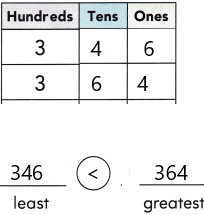Explanation:
346, 364 both the numbers have an equal number of digits, therefore, we will compare the left-most digit of both the numbers.
But the left-most digit is also the same for both numbers, i.e.,3. So, we need to check the second left-most digit.
4 < 6
Therefore,
346 < 364
hence, 346 is smaller than 364.

Math Talk
Mathematical Processes

Describe how the numbers are different.
Answer: By place values, we can differentiate the numbers.
Place value in Maths describes the position or place of a digit in a number. Each digit has a place in a number. When we represent the number in general form, the position of each digit will be expanded. Those positions start from a unit place or we also call it one’s position. The order of place value of digits of a number of right to left is units, tens, hundreds, thousands, ten thousand, a hundred thousand, and so on.
The place value chart is:Model and Draw

You can compare digits to order numbers from least to greatest. Start by looking at the hundreds of place.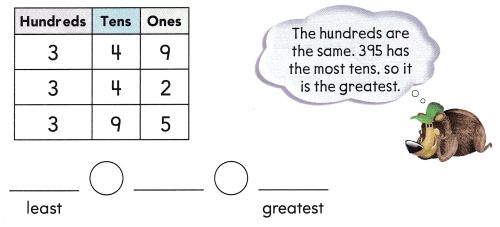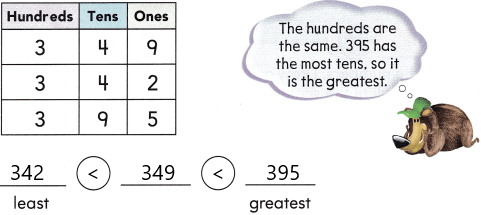Explanation:
The numbers 342, 349, and 395 have an equal number of digits, therefore, we will compare the left-most digit of given numbers.
But the left-most digit is also the same for all numbers, i.e.,3. So, we need to check the second left-most digit.
Now check all the digits present in the tens place: 4, 4, 9
But the left-most digit is also the same for both numbers, i.e.,4. So, we need to check the second left-most digit. Now check with the third number present in the tens place.
4 < 9.
342 < 395
Now check the left-most digits present in the one’s place. Those numbers are 342, 349
2 < 9
Therefore,
342 < 349
By comparing all the numbers we got:
342 < 349 < 395.
Hence, 342 is smaller than 349
349 is smaller than 395.

Share and Show

Compare the numbers. Write them in order from least to greatest.

Question 1.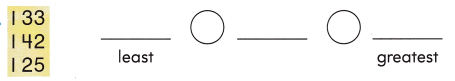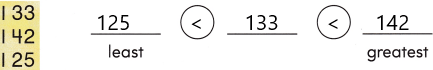Explanation:
125, 133, and 142 the numbers have an equal number of digits, therefore, we will compare the left-most digit of both the numbers.
But the left-most digit is also the same for all the numbers, i.e.,1. So, we need to check the second left-most digit.
2 < 3 < 4
Therefore,
125 < 133 < 142
hence, 125 is smaller than 133
133 is smaller than 142.

Question 2.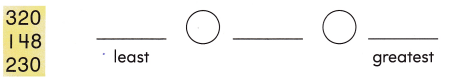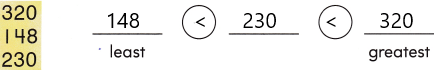Explanation:
Since, all the given numbers, 148, 230, and 320 have three digits, thus we will compare the left-most digit of the given numbers.
1 < 2 < 3
Therefore,
148 < 230 < 320
Hence, 148 is less than 230
230 is less than 320.

Question 3.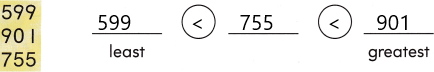Explanation:
Since, all the given numbers, 599, 755, and 901 have three digits, thus we will compare the left-most digit of the given numbers.
5 < 7 < 9
Therefore,
599 < 755 < 901
Hence, 599 is less than 755
755 is less than 901.

Problem Solving

Compare the numbers. Write them in order from least to greatest.

Question 4.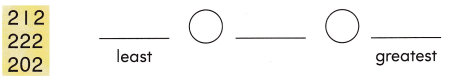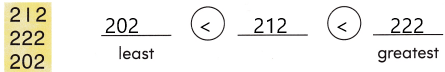Explanation:
1. Since, all the numbers, 212, 222 and 202 are having three digits, thus we will compare the left-most digit of both the numbers.
But the left-most digit is also the same for all the given numbers, i.e.,2. So, we need to check the second left-most digit.
0 < 1 < 2
therefore,
202 < 212 < 222
Hence, 202 is smaller than 212
212 is smaller than 222.

Question 5.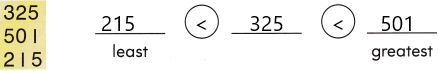Explanation:
1. Since, all the numbers, 325, 501 and 215 are having three digits, thus we will compare the left-most digit of both the numbers.
2. Arrange them in order:
215, 325, 501
2 < 3 < 5
Therefore,
215 < 325 < 501.
Hence, 215 is lesser than 325
325 is less than 501.

Question 6.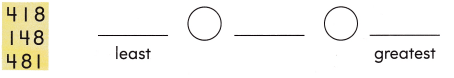Explanation:
1. Since, all the numbers, 418, 148 and 481 are having three digits, thus we will compare the left-most digit of both the numbers.
418 and 481 are having the same digits in hundreds value. So check 1 and 4
1 < 4
148 is less than 418 and 481.
Now check the numbers 418 and 481. But the left-most digit is also the same for both numbers, i.e.,4. So, we need to check the second left-most digit.
1 < 8
therefore,
418 < 481
Hence, 148 < 418 < 481.
148 is smaller than 418
418 is smaller than 481.

Solve. Write or draw to explain.

Question 7.
H.O.T. Multi-Step Mr. Damon has three boxes of paper with 153 sheets, 214 sheets, and 180 sheets. Write the numbers in order from greatest to least. Remember to write comparison symbols.Explanation:
The above-given numbers are 153, 214, 180
These numbers should be written from greatest to least.
arrange them in greatest order: 214, 180, 153
214, 180, 153
Apply the below rule:
This rule is applicable when two numbers are having the same number of digits. In such cases, we need to check the digit at the leftmost place, whichever is greater. Therefore, the number with a greater digit at the leftmost place of the number is greater than the other number.
then we get 214 > 180 > 153.

Question 8.
H.O.T. Nisha wrote these numbers in order from least to greatest. She spills some water on her paper. Write the missing number.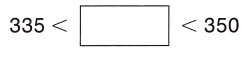Explanation:
Nisha was writing the numbers from least to greatest.
The missing numbers would be starting from 336 to 349.
We can write any number in that above-given figure:
Here we need to write greater than 335 and less than 350.
So I choose the number 345
335 < 345 < 350.
The order is least to greatest.

Question 9.
There are 3 bags with 953 peanuts, 721 peanuts, and 598 peanuts. Which is the greatest number?
(A) 953
(B) 721
(C) 598Explanation:
Option B and option C were wrong.
Option A was showing the correct comparison between the numbers.
Now taking options:
953, 721, 598 all the numbers have an equal number of digits, therefore, we will compare the left-most digit of the given numbers.
9 > 7 > 5
Therefore,
953 > 721 > 598
Hence 953 is greater than other two numbers

Question 10.
Representations Sam writes 631, 321, and 621 on a piece of paper. Which of these numbers is the greatest?
(A) 631
(B) 321
(C) 621Explanation:
Option B and option C were wrong.
Option A was showing the correct comparison between the numbers.
Now taking option all options:
631, 321, 621 all the numbers have an equal number of digits, therefore, we will compare the left-most digit of the given numbers.
But the left-most digit is also the same for the two numbers, i.e.,6. So, we need to check the left-most digit.
6 < 3
Now check the 631 and 621, as we know both the numbers are having the same digits in the place value. So we need to check the left-most digit.
3 > 2
Therefore,
631 > 621 > 321
631 is the greatest number when compared to 621 and 321.
Question 11.
Multi-Step John writes the following numbers on a piece of paper. Which of these numbers is the least?
(A) four hundred twenty-six
(B) 200 + 30 + 9
(C) 371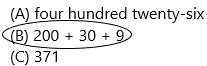Explanation:
We need to write the least number from the above-given options.
Four hundred twenty-six:426
200+30+9:239
another number is 371.
Of the above-given numbers, 239 is the least number.

Question 12.
TEXAS Test Prep Which of these is true?
(A) 161 < 149 < 152
(B) 161 < 152 < 149
(C) 149 < 152 < 161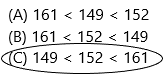Explanation:
Option A and option B were wrong.
Option C was showing the correct comparison between the numbers.
Now taking option C:
149, 152, 161 all the numbers have an equal number of digits, therefore, we will compare the left-most digit of the given numbers.
But the left-most digit is also the same for the given numbers, i.e.,1. So, we need to check the second left-most digit.
4 < 5 < 6
Therefore,
149 < 152 < 161
Hence 149 is smaller than 152
152 smaller than 161.

TAKE HOME ACTIVITY • Ask your child to show how he or she solved an exercise in the lesson.
– The three comparison symbols are less than (<), greater than (>) and equal to (=).
– The “equal to” symbol is used to represent two equal numbers or quantities, such as:
– 3 = 3 or 10 – 7 = 3
– The “greater than” symbol is used to show if a number is greater than the other number. For example,
10 > 3 [10 is greater than 3]
– The “less than” symbol is used when a number or a quantity is less than the other. For example,
9 < 11 [9 is less than 11].
Use of less than and greater than:
– The less than and greater than symbols are used based on two numbers given to compare.
– A bigger number greater than a smaller number is represented as:
Bigger Number > Smaller Number
– A smaller number less than a greater number is represented as:
Smaller Number < Greater Number.

Scroll to Top